#DAY 20
0

### How to Train Your Model 訓模高手：我的 Tensorflow 個人使用經驗系列文系列 第 20 篇

``````global_step = global_step = tf.train.get_or_create_global_step()

x = tf.placeholder(shape=[None, 2], dtype=tf.float32, name='x')
y = tf.placeholder(shape=[None], dtype=tf.int32, name='y')

with tf.variable_scope('backend'):
net = tf.layers.dense(x, 64, activation=tf.nn.relu6,
kernel_initializer=WEIGHT_INIT,
bias_initializer=BIAS_INIT,
kernel_regularizer=REGULARIZER,
bias_regularizer=REGULARIZER,
name='dense_1')
net = tf.layers.dense(net, 64, activation=tf.nn.relu6,
kernel_initializer=WEIGHT_INIT,
bias_initializer=BIAS_INIT,
kernel_regularizer=REGULARIZER,
bias_regularizer=REGULARIZER,
name='dense_2')
logits = tf.layers.dense(net, 2, kernel_initializer=WEIGHT_INIT,
bias_initializer=BIAS_INIT,
kernel_regularizer=REGULARIZER,
bias_regularizer=REGULARIZER,
name='final_dense')

loss = tf.reduce_mean(
tf.nn.sparse_softmax_cross_entropy_with_logits(
logits=logits, labels=y), name='inference_loss')

``````

``````def get_optimizer(opt_type):
if opt_type == 'gd':
if opt_type == 'momentum':
return tf.train.MomentumOptimizer(learning_rate=0.1, momentum=0.9)
if opt_type == 'rmsp':
return tf.train.RMSPropOptimizer(learning_rate=0.1, decay=0.9, momentum=0)

``````

``````opt = get_optimizer(opt_type)

``````

``````def get_xor_data():
x = (np.random.rand(16, 2) - 0.5) * 2
y = [0 if 0 < x1 * x2 else 1 for x1, x2 in x]

return x, y
``````

``````with tf.Session() as sess:
sess.run(tf.global_variables_initializer())
sess.run(tf.local_variables_initializer())

start = timeit.default_timer()
for _ in range(0, 100):
x_v, y_v = get_xor_data()
_, count = sess.run([train_op, global_step], feed_dict={x: x_v, y: y_v})

print(f'iter: {count}')

print(f'done. cost {timeit.default_timer() - start} sec.')
``````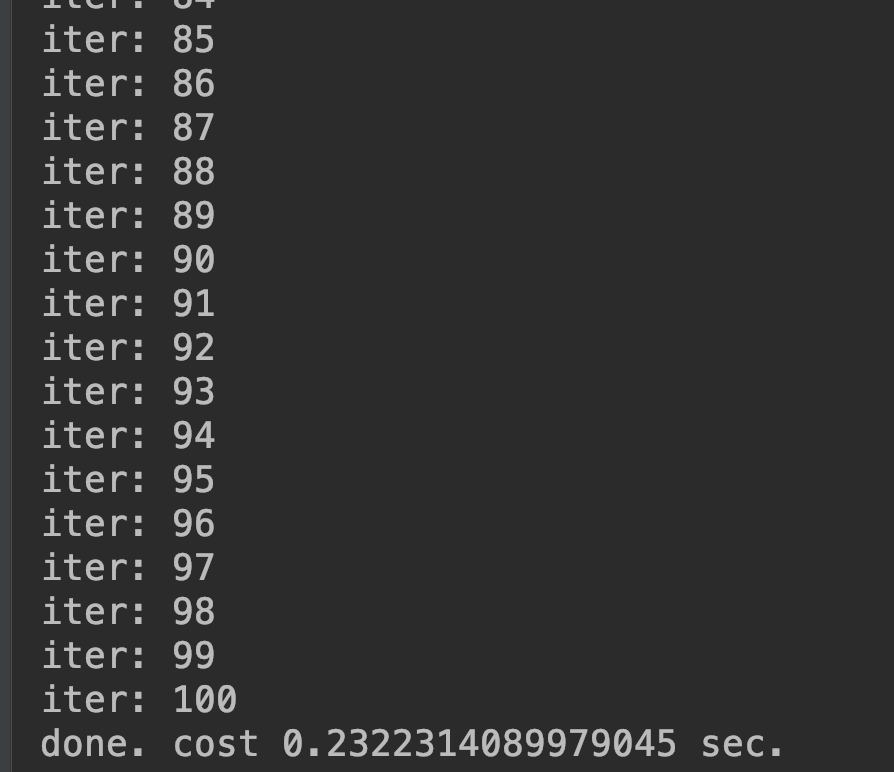tensorflow 幫我們做了那麼多種優化器，用起來是很方便，但是你真的懂每個優化器使用時機嗎？沒錯，這次我也想挑戰以自己的話來解釋這幾種優化器的差異！

``````tf.train.GradientDescentOptimizer(learning_rate=0.1)
``````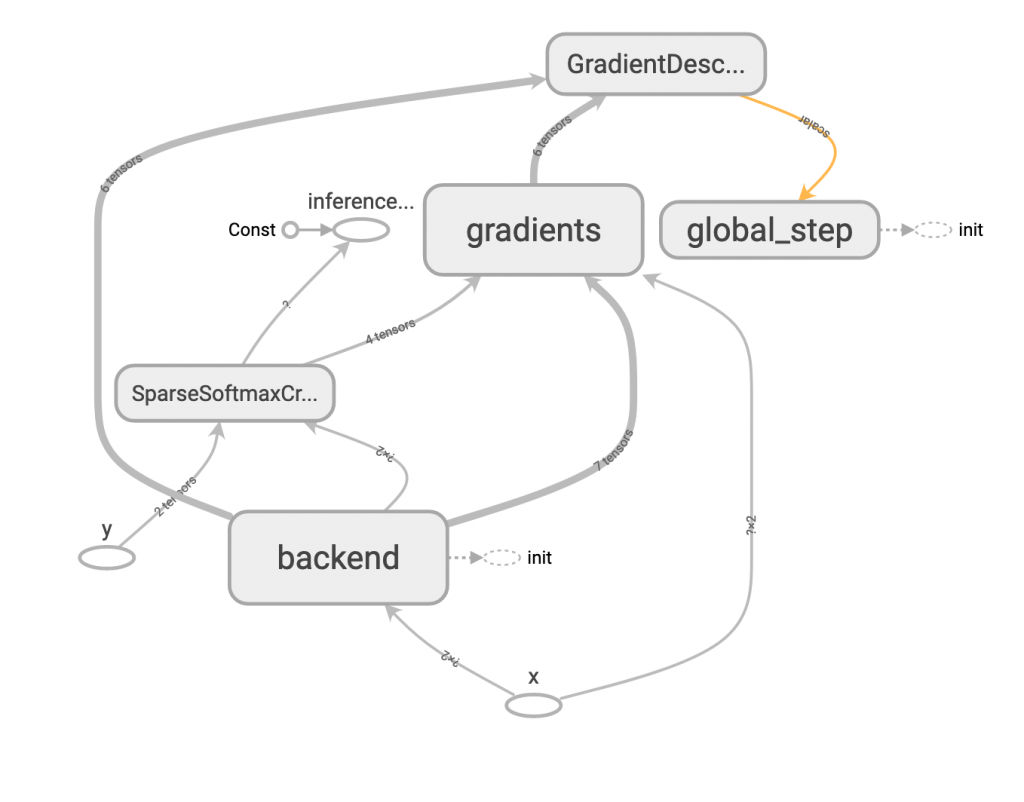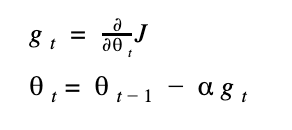``````tf.train.MomentumOptimizer(learning_rate=0.1, momentum=0.9)
``````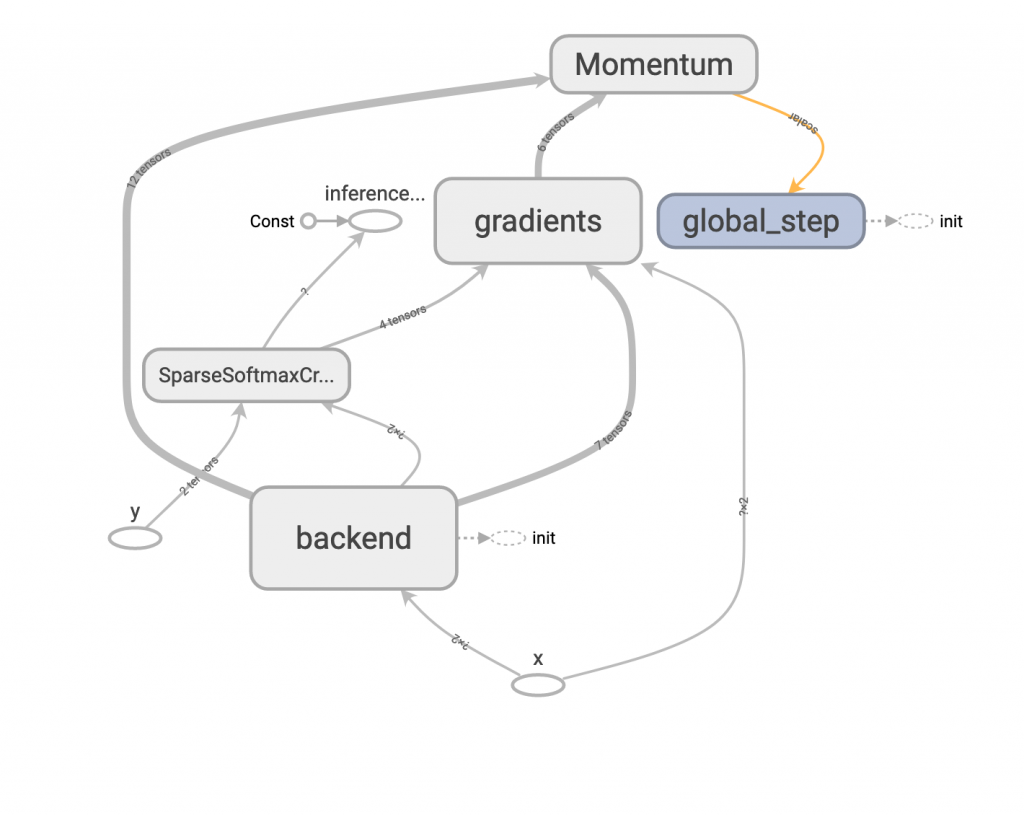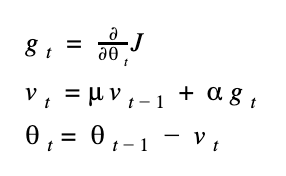mu (很像 u 的符號) 表示 momentum 的參數值，v 表示目前更新值的大小，因此當模型開始訓練時，每次的更新量會被之前的更新量牽制，比較不容易忽大忽小，以增加穩定性。

``````tf.train.AdagradOptimizer(learning_rate=0.1, initial_accumulator_value=0.1)
``````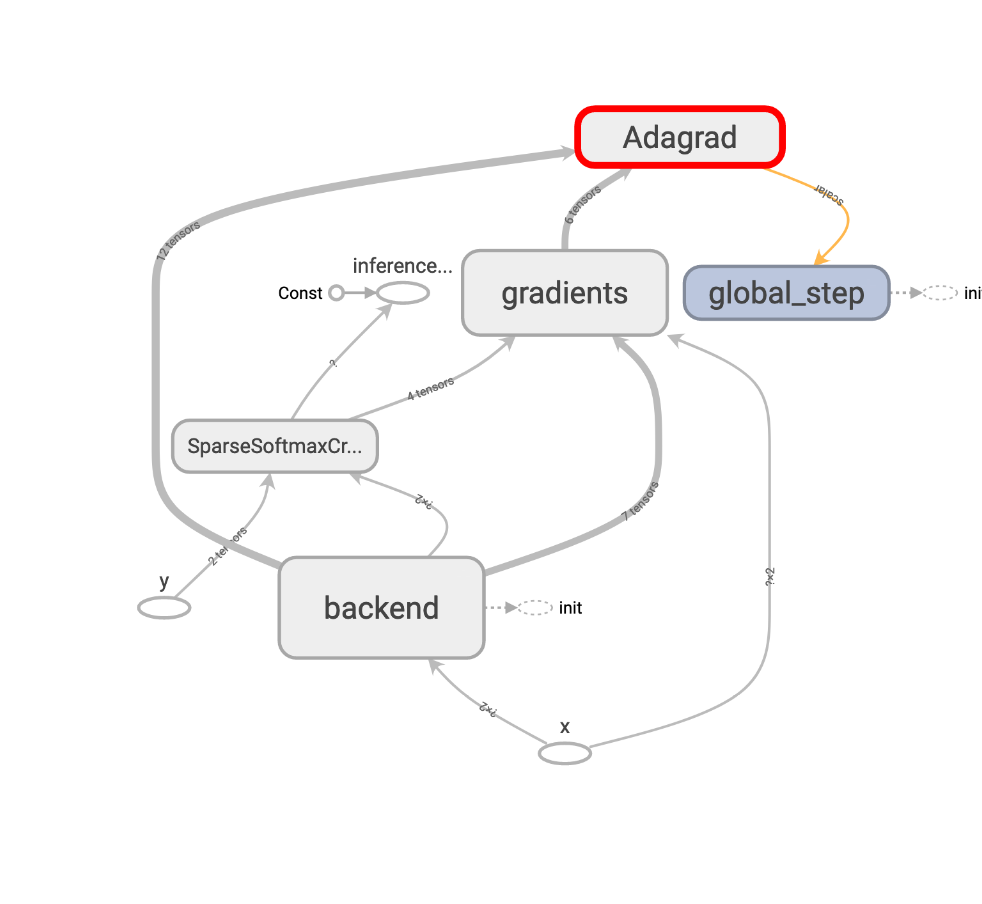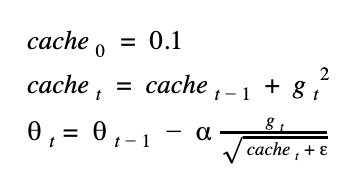``````tf.train.RMSPropOptimizer(learning_rate=0.1, decay=0.9, momentum=0)
``````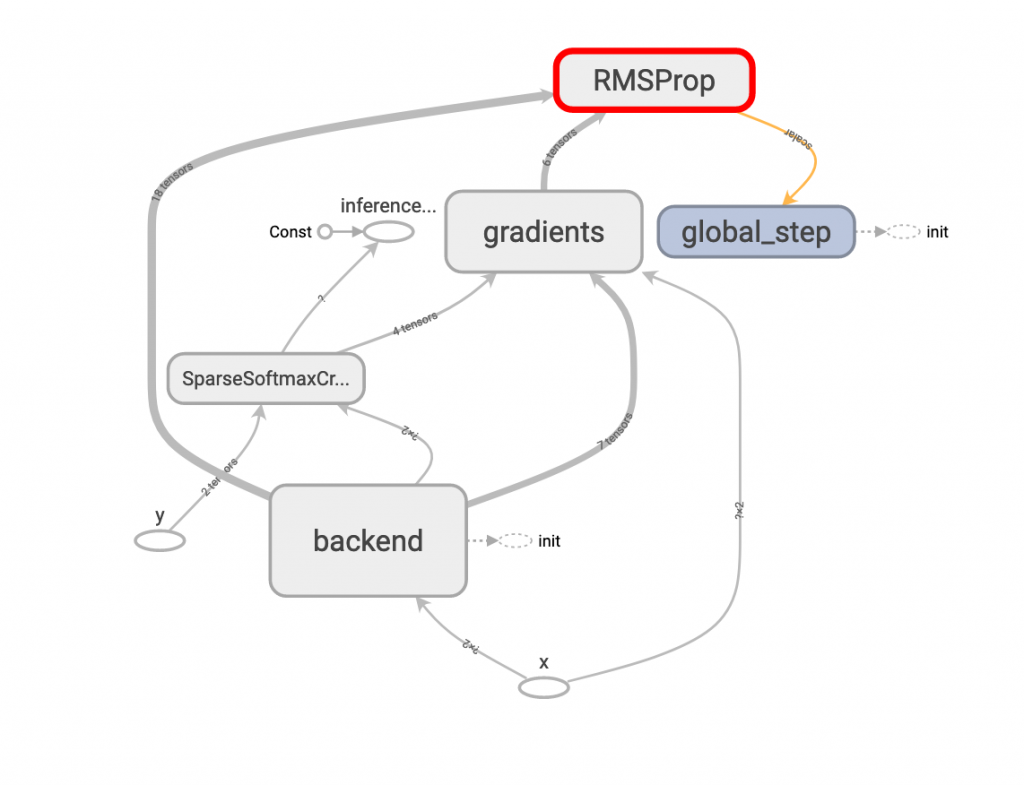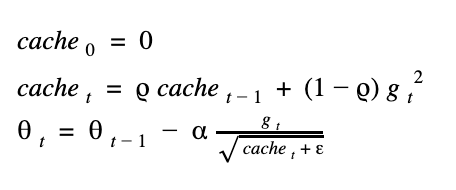``````tf.train.AdamOptimizer(learning_rate=0.1, beta1=0.9, beta2=0.99)
``````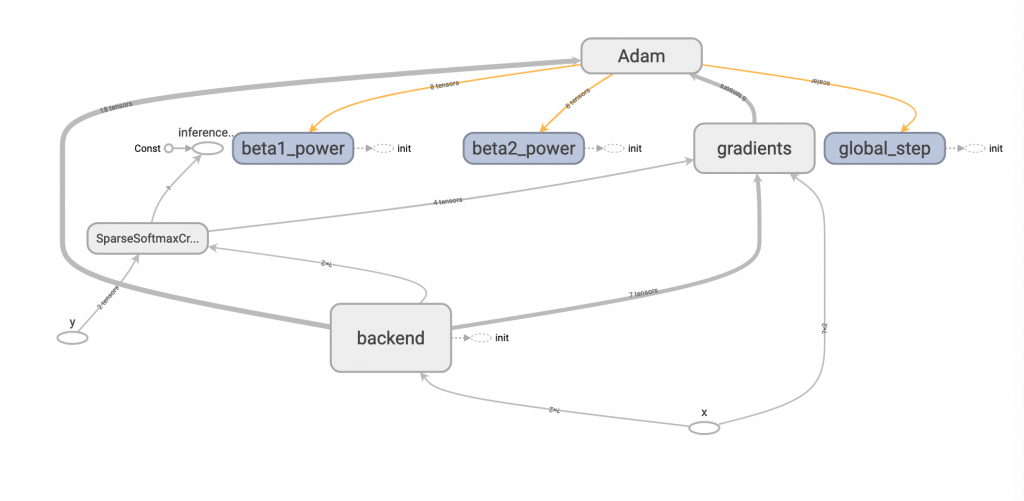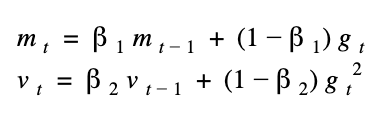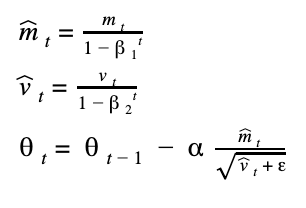github原始碼